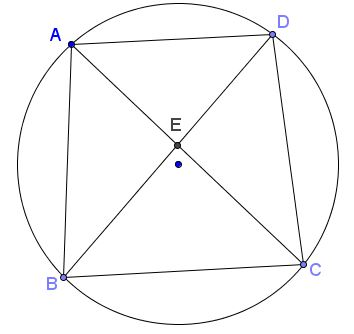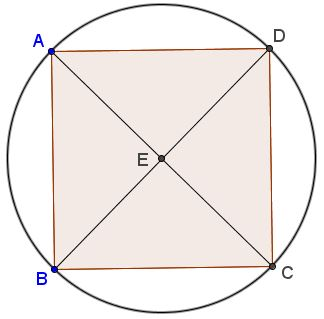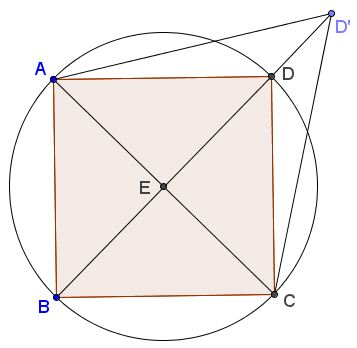# An Identity in (Cyclic) Quadrilaterals

### Problem

Let $ABCD$ be a cyclic quadrilateral; $E$ the intersection of the diagonals.Then

$\displaystyle\frac{AE}{CE}=\frac{AB}{BC}\cdot\frac{AD}{CD}.$

Introduce the angles as shown in the diagram below$I shall apply the Law of Sines repeatedly in several triangles:$\begin{align}\displaystyle \Delta ABE: & \frac{AE}{BE}=\frac{\sin\delta}{\sin\beta},\\ \Delta BCE: & \frac{BE}{CE}=\frac{\sin\gamma}{\sin\alpha},\\ \Delta ABC: & \frac{AB}{BC}=\frac{\sin\gamma}{\sin\beta},\\ \Delta ACD: & \frac{AD}{CD}=\frac{\sin\delta}{\sin\alpha}. \end{align}$Multiplying the first and the second pair separately yields:$\displaystyle \frac{AE}{CE}=\frac{AE}{BE}\frac{BE}{CE}=\frac{\sin\delta}{\sin\beta}\frac{\sin\gamma}{\sin\alpha}=\frac{AB}{BC}\frac{AD}{CD},$which proves the statement. ### Observation The identity$\displaystyle\frac{AE}{CE}=\frac{AB}{BC}\cdot\frac{AD}{CD}$is a necessary but not a sufficient condition for the quadrilateral$ABCD$to be cyclic. The clue why this be so stems from its lack of symmetry. It compares poorly with the symmetry exhibited by Ptolemy's theorem that naturally comes to mind. It is easy to exploit this lack of symmetry. Start with a square which is naturally cyclic:Now, extend vertex$D$along the diagonal$BD$to a new position$D'.$We still have$\displaystyle\frac{AE}{CE}=\frac{AB}{BC}\cdot\frac{AD'}{CD'}$(because$\displaystyle\frac{AD}{CD}=1=\frac{AD'}{CD'})$but the quadrilateral$ABCD'\$ is no longer cyclic.### Acknowledgment

The problem has been posted by Dao Thanh Oai (Vietnam) at the CutTheKnotMath facebook page.